Question

Draw the RTL schematic of the hardware that will be synthesized for the VHDL code below.

entity unknown is

port (x: in std_logic_vector(7 downto 0);

op: in std_logic_vector(1 downto 0);

clk: in std_logic;

f: out std_logic_vector(7 downto 0));

end entity.

architecture arch of unknown is

signal a, b, c, d: std_logic_vector(7 downto 0);

begin

d <= x;

process (clk)

begin

if (rising_edge(clk)) then

a <= b;

b <= c + a;

c <= d;

if (op = “00”) then

f <= a;

elsif (op =”01”) then

f <= a + b;

elsif (op=”10”) then

f <= b + c;

else

f <= d + c;

end if;

end process;

end architecture;

We need at least 10 more requests to produce the answer.

0 / 10 have requested this problem solution

The more requests, the faster the answer.

All students who have requested the answer will be notified once they are available.

#### Earn Coins

Coins can be redeemed for fabulous gifts.

Similar Homework Help Questions
• ### Write a test bench for the following VHDL code -------------------------------------------------------------------------------------------------------------------------------- LIBRARY ieee ; USE ieee.std_logic_1164.all ;...

Write a test bench for the following VHDL code -------------------------------------------------------------------------------------------------------------------------------- LIBRARY ieee ; USE ieee.std_logic_1164.all ; ENTITY registern IS GENERIC (N: INTEGER :=4);    -- INTEGER=32, 16, ….. PORT (D           : IN STD_LOGIC_VECTOR (N-1 downto 0); clk, reset, Load   : IN      STD_LOGIC ; Q           : OUT   STD_LOGIC_VECTOR (N-1 downto 0 )) ; END registern; ARCHITECTURE behavior OF registern IS BEGIN PROCESS (clk) BEGIN IF clk' EVENT AND clk='1' THEN IF (reset ='0') THEN    --synchronous reset Q<=(OTHERS=>’0’); ELSIF (L ='0') THEN Q<=D;...

• ### QUESTION 1 Complete the following peice of VHDL code with the necessary VHDL statements for a...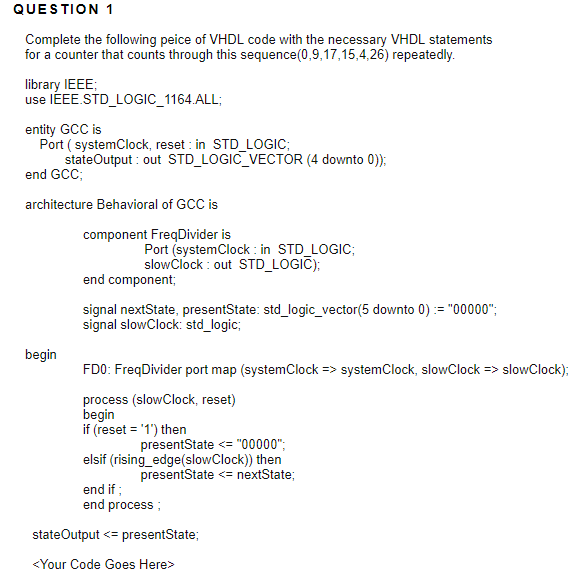QUESTION 1 Complete the following peice of VHDL code with the necessary VHDL statements for a counter that counts through this sequence(0,9,17,15,4,26) repeatedly. library IEEE use IEEE.STD_LOGIC_1164 ALL entity GCC is Port ( systemClock, reset in STD_LOGIC end GCC architecture Behavioral of GCC is stateOutput out STD LOGIC_ VECTOR (4 downto 0)) component FreqDivider is Port (systemClock in STD_LOGIC; slowClock: out STD LOGIC); end component, signal nextState, presentState: std_logic_vector(5 downto 0) := "00000"; signal slowClock: std_logic begin FD0: FreqDivider port...

• ### Create a new architecture for ripple_counter (below) called beh_mod10cnt that changes the behavior to a modulo-10...

Create a new architecture for ripple_counter (below) called beh_mod10cnt that changes the behavior to a modulo-10 ripple counter. The ripple counter entity entity ripple_counter is generic (n : natural := 4); port ( clk : in std_logic; clear : in std_logic; dout : out std_logic_vector(n-1 downto 0) ); end ripple_counter; The ripple counter architecture architecture arch_rtl of ripple_counter is signal clk_i : std_logic_vector(n-1 downto 0); signal q_i : std_logic_vector(n-1 downto 0); begin clk_i(0) <= clk; clk_i(n-1 downto 1) <= q_i(n-2...

• ### Write a VHDL code using processes for the following logic circuit which include a shift register...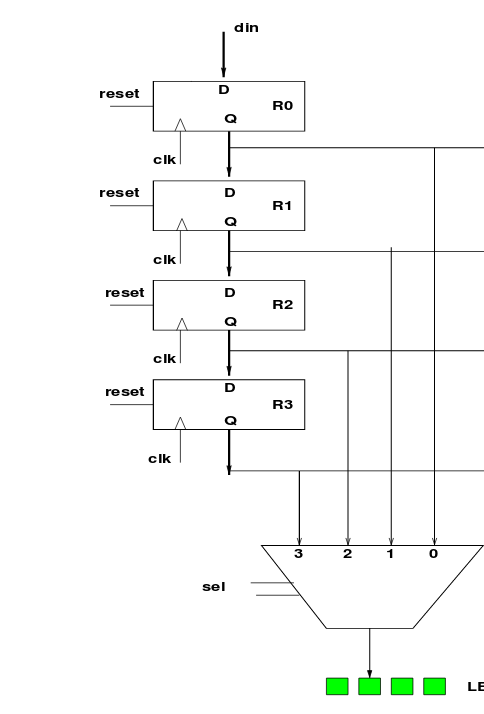Write a VHDL code using processes for the following logic circuit which include a shift register and 4x1 multiplexer. Use the entity below.              entity registers_min_max is port( din   : in std_logic_vector(3 downto 0);       reset : in std_logic;       clk   : in std_logic;       sel   : in std_logic_vector(1 downto 0);       reg_out : out std_logic_vector(3 downto 0)); end registers_min_max; din reset RO clk reset R1 A C clk reset R2 clk reset R3 clk 3 0 sel LE

• ### 8.(5 points).There is an error in following VHDL code. Find the error and correct (only that...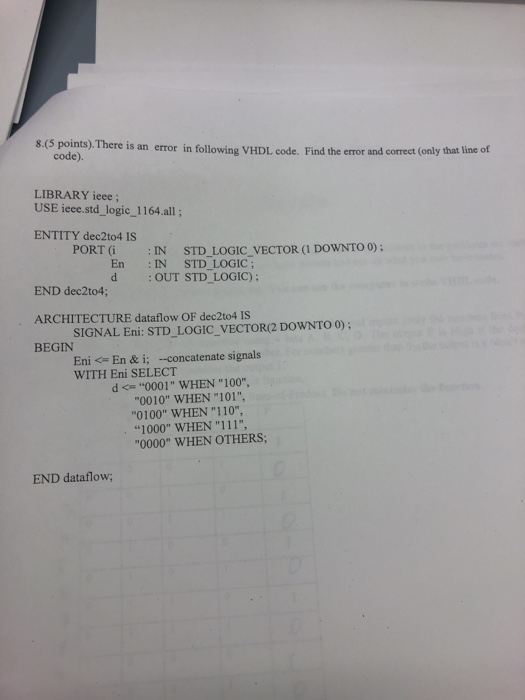8.(5 points).There is an error in following VHDL code. Find the error and correct (only that line of code). LIBRARY ieee; USE ieee.std_logic_1164.all; ENTITY dec2to4 IS PORT (i IN STD LOGIC VECTOR (1 DOWNTO 0); : En IN STD_LOGIC; d OUT STD LOGIC); END dec2to4; ARCHITECTURE dataflow OF dec2to4 IS BEGIN SIGNAL Eni: STD_LOGIC_VECTOR(2 DOWNTO 0); Eni <= En & i; -concatenate signals WITH Eni SELECT d <"0001" WHEN "100" "0010" WHEN "101", "0100" WHEN "110", "1000" WHEN "111", 0000"...

• ### car taillights help with code

x.øi5nt face="Times New Roman" size="3">I am trying to do a T-Bird tailight function using VHDL using model sim. I have been able to get the emergency (Hazard)and Left and right turn to work but can not get the brake light function to work. below is my codewhich turns on the hazard and turn signals correctly (on sparta s3 board or Model sim simulation)so what i want to do is "When the brakes are on, andwhen the hazard is on, it...

• ### Some questions may require well bulum 1. HDL stands for? a. Hardware Design Language b. Hardware...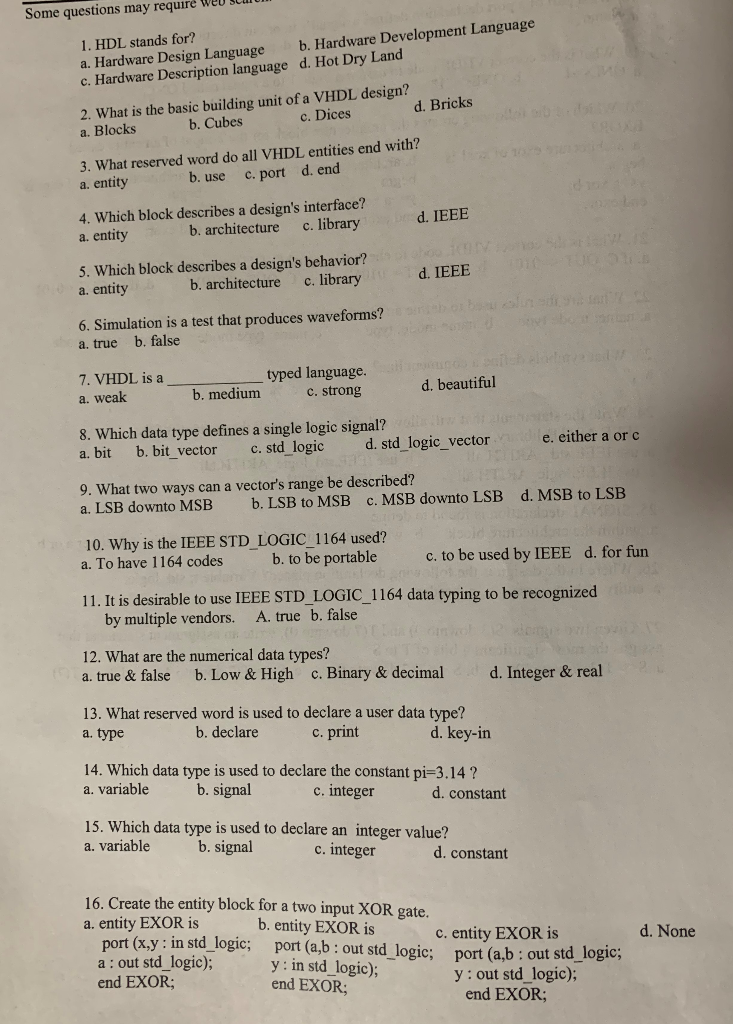Some questions may require well bulum 1. HDL stands for? a. Hardware Design Language b. Hardware Development Language c. Hardware Description language d. Hot Dry Land 2. What is the basic building unit of a VHDL design? a. Blocks b. Cubes c . Dices d. Bricks 3. What reserved word do all VHDL entities end with? a. entity b. use c. port d. end d. IEEE 4. Which block describes a design's interface? a. entity b. architecture c. library 5....

• ### Name: ·5. (10 lts) Find and correct errors in the following VHDL ed. IEEE ; library use IEEE . STD LOGIC-1104 . all...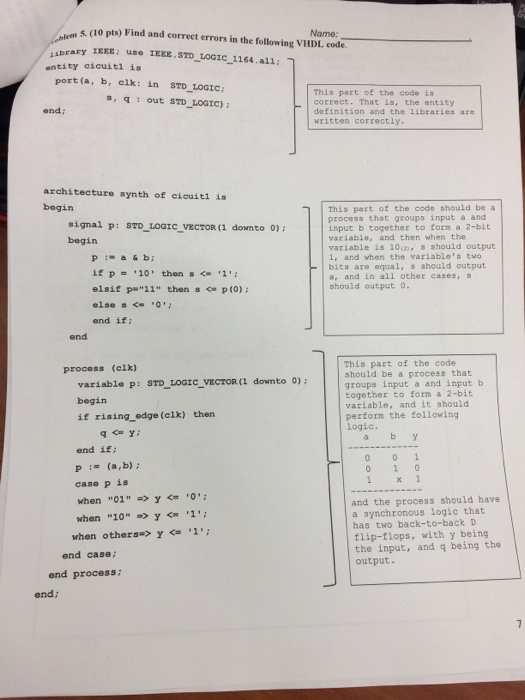Name: ·5. (10 lts) Find and correct errors in the following VHDL ed. IEEE ; library use IEEE . STD LOGIC-1104 . all; entity cicuitl is port (a, b, elk: in STD_LOGIC: This part of the code its correct.That is, the entity definition and the 1ibraries are written correctly S out STD LOGIC) ond; architecture synth of eicuiti is begin This part of the code ธhould be a process that groups input a and input b together to forn a...

• ### draw a block diagram of the circuit represented by the vhdl code listed below. be sure...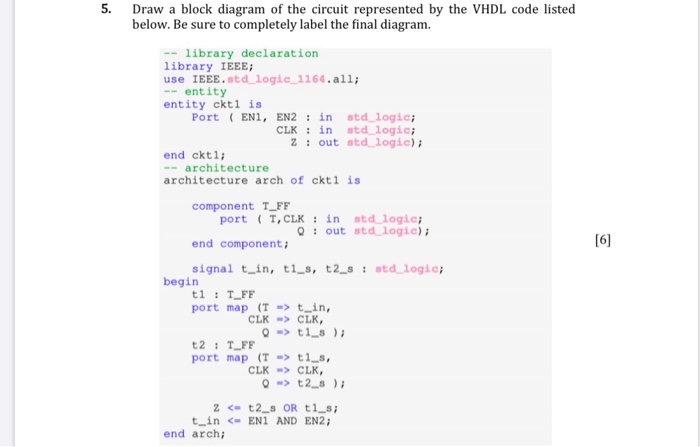draw a block diagram of the circuit represented by the vhdl code listed below. be sure to completely label the final diagram Draw a block diagram of the circuit represented by the VHDL code listed below. Be sure to completely label the final diagram. -- library declaration library IEEE; use IEEE.std logic 1164.all; -- entity entity ckti is Port (EN, EN2 : in std logic; CLK : in std logic; Z! out std logie); end ckt1; -- architecture architecture arch...

• ### in vhdl Manchester code is a coding scheme used to represent a bit in a data...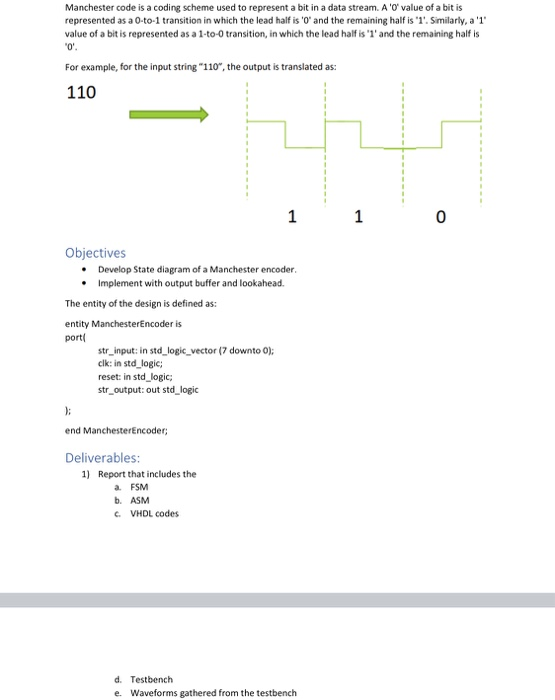in vhdl Manchester code is a coding scheme used to represent a bit in a data stream. A 'O' value of a bit is represented as a 0-to-1 transition in which the lead half is 'O' and the remaining half is '1'. Similarly, a '1' value of a bit is represented as a 1-to-transition, in which the lead half is 'l' and the remaining half is 0 For example, for the input string "110", the output is translated as: 110...